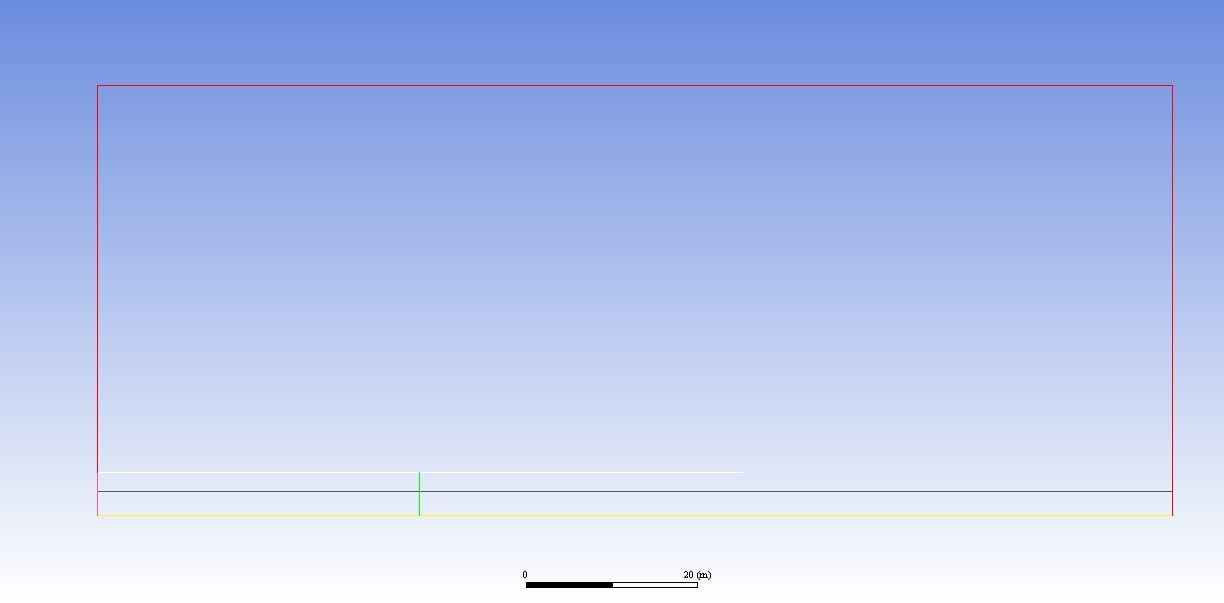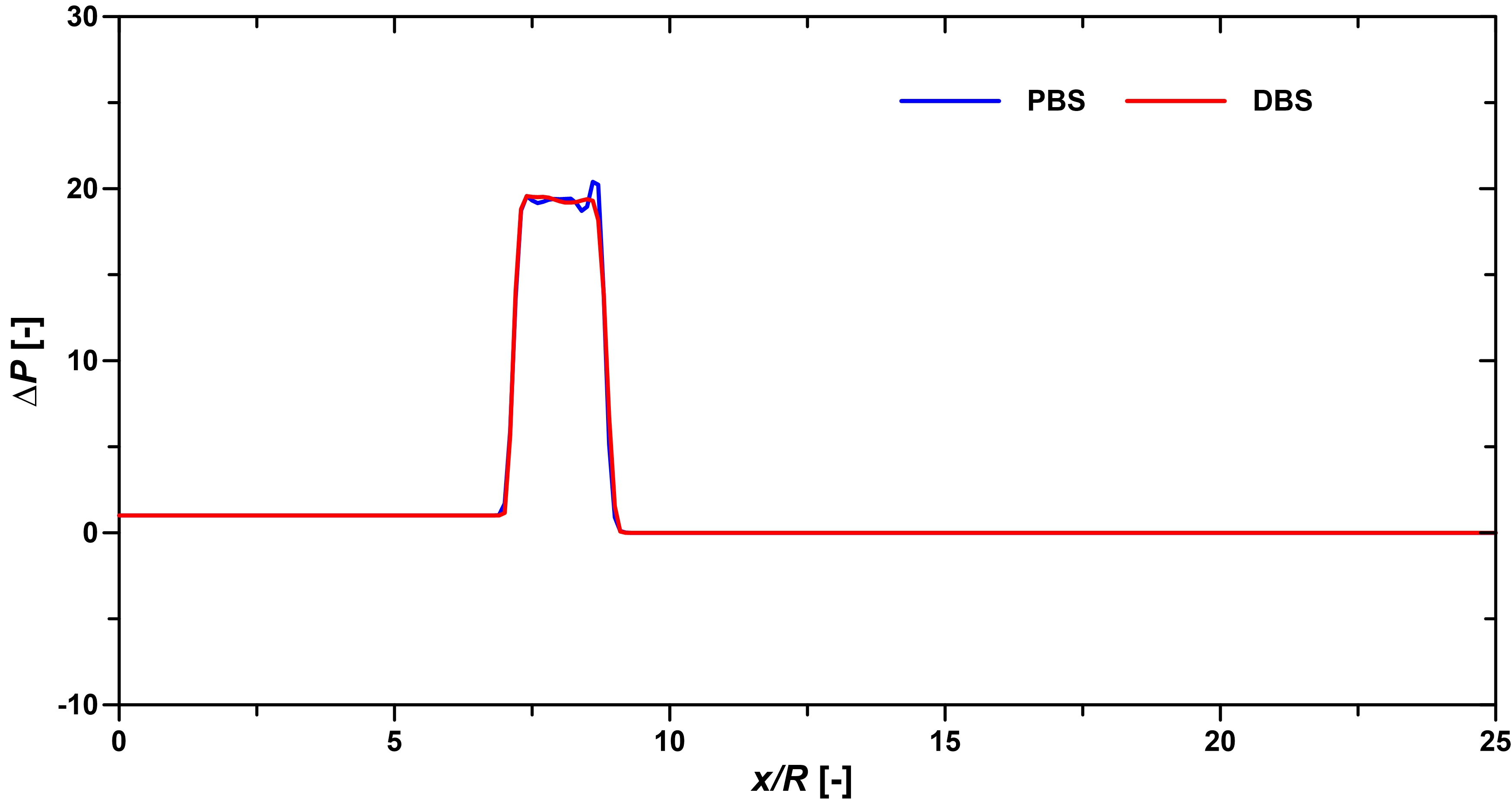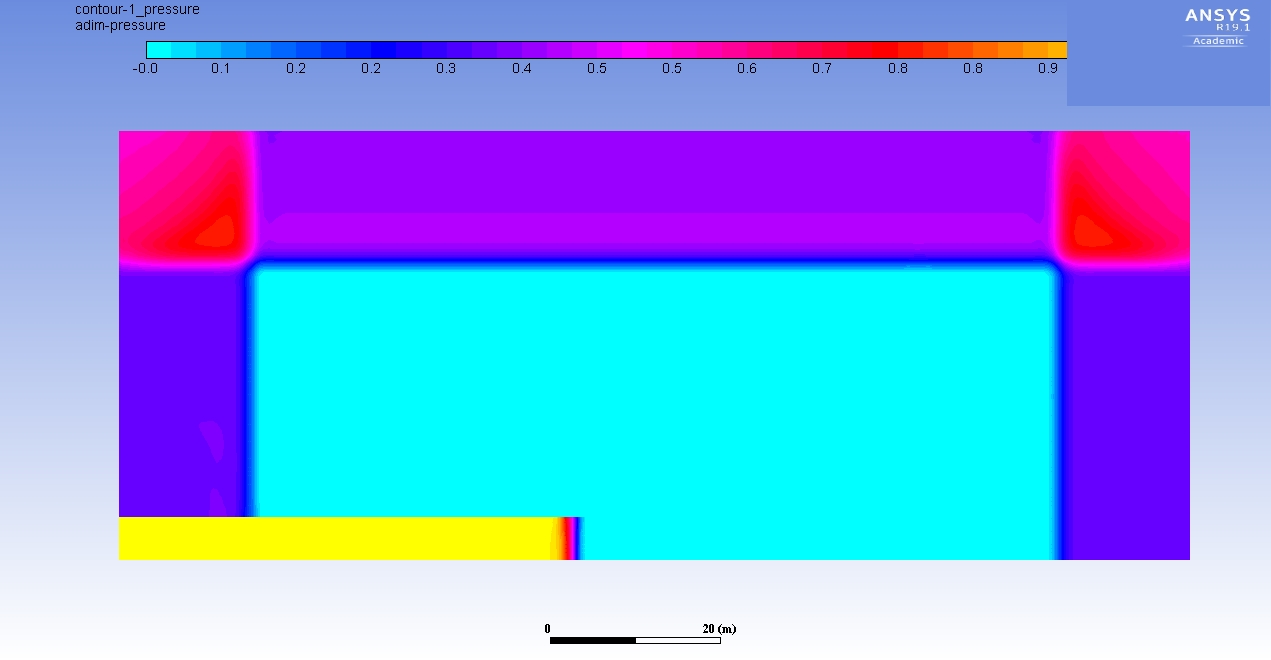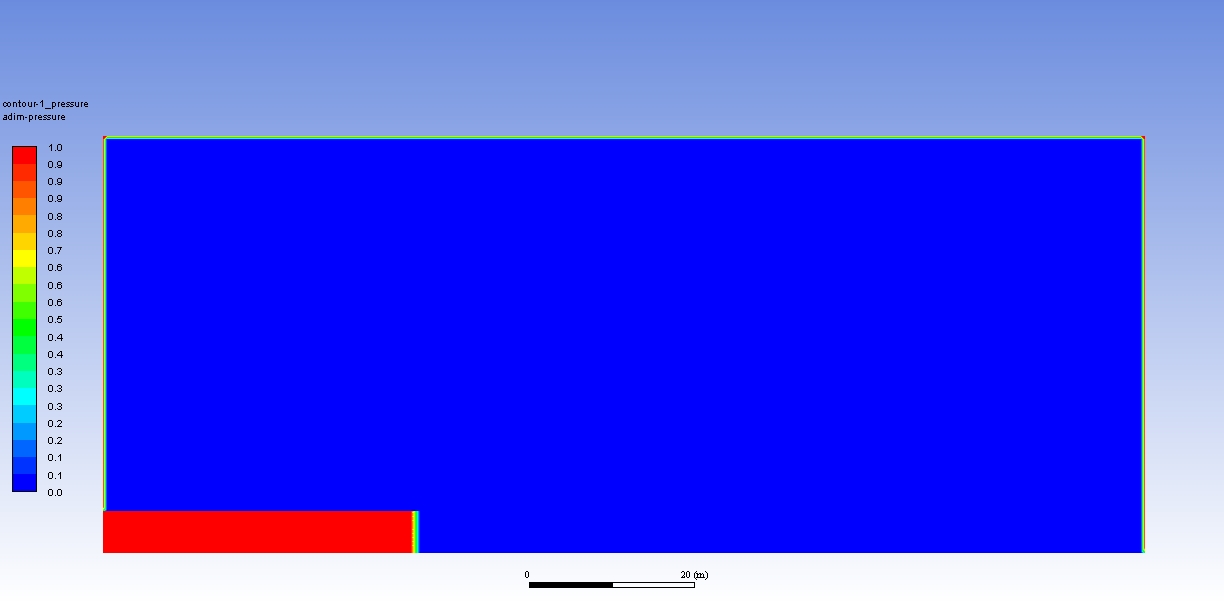## Fluids

•egorbena
Subscriber

Hi,

I'm trying to simulate a shock-wave travelling in a tunnel and expansion to the atmosphere (see attached figure showing the domain and location of the shock-wave). I'm assessing the results obtained using a Pressure Based Solver (PBS) and Density Based Solver (DBS) when the shock-wave is still in the tunnel. As you can see in the attached graph (taken at the profile marked in black in the domain) both solvers provide almost similar results. At the plateau of the shock-wave, the PBS produces larger pressure oscillations than the DBS. My questions are:

• Why are happening these oscillations?

• Why the solvers produce different results?

• Based on this result, what solver should I use and why?

Thank you.

Kind regards,

EduardoTunnel wall "wall" (white line); domain outlet "pressure outlet" (red lines); axisymmetric "axis" (yellow line); shock-wave position (green line); and longitudinal profile where results are taken (black line).•DrAmine
Ansys Employee

The solver produce different results as they are different solver using different numerics and primitive variables.

In both methods the velocity field is gained from the momentum equations. In the density-based approach, the continuity equation is used to obtain the density field while the pressure field is determined from the equation of state.On the other hand, in the pressure-based approach, the pressure field is extracted by solving a pressure or pressure correction equation which is obtained by manipulating continuity and momentum equations.

The pressure-based solver traditionally has been used for incompressible and weak compressible flows. The density-based approach, on the other hand, was originally designed for high-speed compressible flows. But now both can be used for almost all flows.

You need to tell us more about the models and numerics used in each solver. Seems like the PBS solution is suffering from some wiggles.

•egorbena
Subscriber

Hi Amine,

In both models I used and inviscid unsteady condition. In the DBS, I used a coupled algorithm with 2nd order spatial discretization and 2nd order implicit temporal discretization. In the PBS, I used a Roe flux-difference-splitting, 2nd order spatial discretization and 2nd order implicit temporal discretization.

Kind regards,

Egorbena

•DrAmine
Ansys Employee
Check with bounded for pressure based it it helps.
•egorbena
Subscriber

Dear all,

I tried to solve the above transient problem using two different conditions for the outlet boundaries to assess the propagation of the shock-wave outside the tunnel. These are:

• Pressure outlet using standard density based solver.

• Pressure outlet using density based solver with acoustic wave model - non reflecting (to avoid unreal reflection of the shock-wave from the outlet boundaries).

In the first approach, the shock wave is reflected at the boundaries. In the second approach, I wasn't able to run the simulation from t = 0, when doing so, looks like a high pressure is entering from the outlets (see attached figure). Instead, if I run the simulation for some time-states with the pressure outlet using standard density based and then I switch to the acoustic wave model - non reflecting condition, the result is fine.

What setting should I use to be able to run a DBS-AWMNR from t = 0?

Thank you.

Kind regards,

Eduardo•DrAmine
Ansys Employee
Which equation is starring diverging? You can reduce density URF and tine step size. I will stick to the approach you followed.
•egorbena
Subscriber

Dear Amine,

The divergence occurs when I use a density based solver with pressure outlet boundaries with the acoustic wave model - non reflecting condition is enabled and the simulation is run from t = 0.

The settings of the model are:

General:

Density based solver; Absolute velocity formulation; Transient; 2D Space: Axisymmetric.

Models:

Energy: on; Viscous: laminar.

Materials:

Air, density: ideal-gas; thermal conductivity and viscosity: kinetic-theory.

Boundary conditions:

Intel > Pressure inlet; Gauge total pressure: 105000 Pa; Normal to boundary.

Outlet > Pressure outlet; Gauge pressure: 100000 Pa; Backflow direction: Normal to boundary; Acoustic wave model: non reflecting, Exit pressure specification: pressure at infinity.

Solution methods:

Formulation: implicit; Flux-type: Roe-FDS; Spatial discretization > Gradient: Least squares cell based, Flow: 2nd Order Upwind; Transient formulation: 2nd Order implicit.

Run calculation:

Time step size (s): 0.000293916

•DrAmine
Ansys Employee

But it is running after you used the solution of the pressure outlet with any additional treatment, right?

•egorbena
Subscriber

Yes, it is running correctly after I run the model for a few time steps using the standard pressure outlet and then I switch to the acoustic wave model with non reflecting condition. I was wandering why I couldn't run the acoustic wave model with non reflecting condition from t = 0.

Kind regards,

Eduardo

•DrAmine
Ansys Employee
Start-up problems might be the reason. As it is working you can neglect the first time step. If you do not like that try reducing tine step size and increase the number of iterations per time step at the beginning ( at first).
•egorbena
Subscriber

Thank you Amine!

•DrAmine
Ansys Employee
Reduce CFL at start-up can also help.
•DrAmine
Ansys Employee
•egorbena
Subscriber

The initialization is done in the following manner:

• Standard initialization; Relative to cell zone; Gauge pressure: 105000 Pa; Axial velocity: 11.89889 m/s; Temperature: 292.0438 K.

• For the rest of the fluid domain I do a patch with: Pressure: 100000 Pa; Axial velocity: 0; Radial velocity: 0; Temperature 288 K.

See the attached figure showing the dimensionless contour plot of pressure after initialization. The red contour will represent a P = 105000 Pa, and the blue contour a pressure of 100000 Pa.

Kind regards,